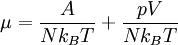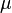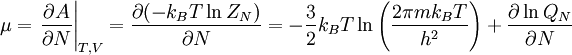# Talk:Chemical potential$\mu=\frac{A}{Nk_B T} + \frac{pV}{Nk_BT}$$\mu$ has wrong dimensionality. Truly dimensionality of$\mu$ is dimensionality of energy.--Sergius (talk) 11:49, 23 August 2013 (CEST)$\mu= \left. \frac{\partial A}{\partial N}\right\vert_{T,V}=\frac{\partial (-k_B T \ln Z_N)}{\partial N} = -\frac{3}{2} k_BT \ln \left(\frac{2\pi m k_BT}{h^2}\right) + \frac{\partial \ln Q_N}{\partial N}$